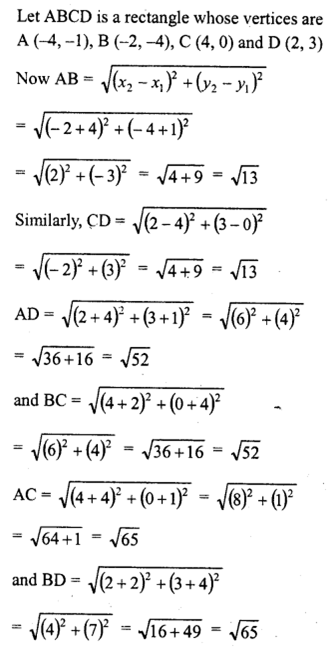• +91 9971497814
• info@interviewmaterial.com

# RD Chapter 14- Co-ordinate Geometry Ex-14.2 Interview Questions Answers

### Related Subjects

Question 1 :
Find the distance between the following pair of points :
(i) (-6, 7) and (-1, -5)
(ii) (a + b, b + c) and (a – b, c – b)
(iii) (a sin α, -b cos α) and (-a cos α, -b sin α)
(iv) (a, 0) and (0, b)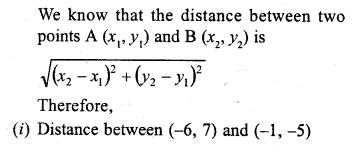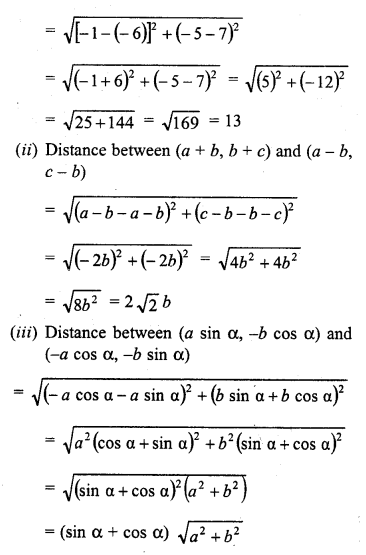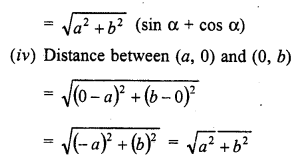Question 2 : Find the value of a when the distance between the points (3, a) and (4, 1) is √10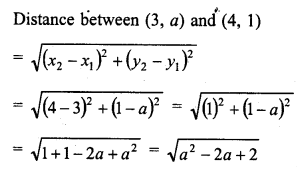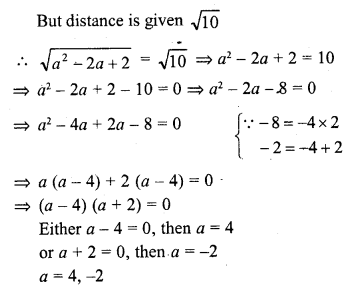Question 3 : If the points (2, 1) and (1, -2) are equidistant from the point (x, y), show that x + 3y = 0.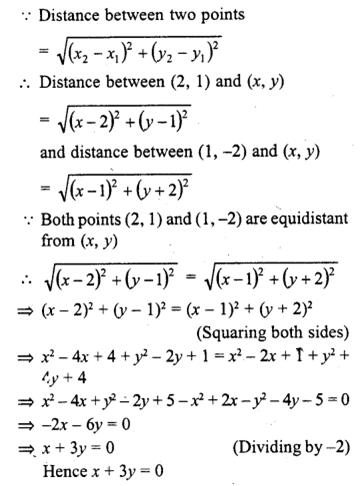Question 4 : Find the values of x, y if the distances of the point (x, y) from (-3, 0) as well as from (3, 0) are 4.

Answer 4 : Distance between (x, y) and (-3, 0) is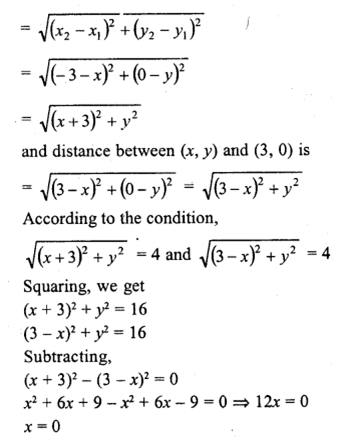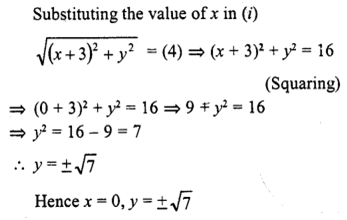Question 5 : The length of a line segment is of 10 units and the coordinates of one end-point are (2, -3). If the abscissa of the other end is 10, find the ordinate of the other end.

Answer 5 : Let the ordinate of other end by y, then The distance between (2, -3) and (10, y) is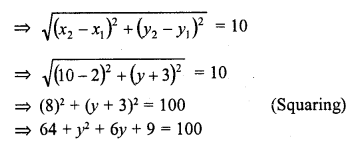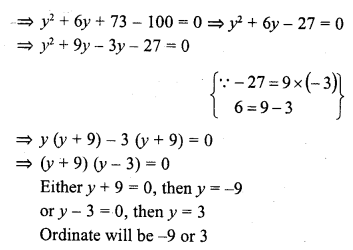Question 6 : Show that the points (-4, -1), (-2, -4), (4, 0) and (2, 3) are the vertices points of a rectangle. (C.B.S.E. 2006C)